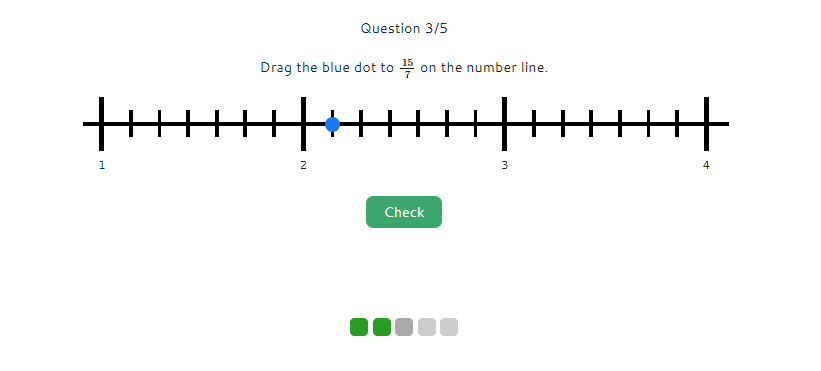MM Practice
×
Multiplication
Division
Place Value
Fractions & Decimals
Measurement
Statistics & Probability
Pre-Algebra
Money
Kindergarten
Geometry

# Fractions on a number lineOnline practice for grades 3-5

Practice marking fractions on a number line with this interactive exercise. You will be shown a number line, and you drag a dot to the correct place to represent the given fraction (standard CCSS.3.NF.A.2).

You can choose to work with proper or improper fractions and/or mixed numbers. Proper fractions are the easiest, whereas placing an improper fraction on a number line probably requires you to change it to a mixed number first. You also have the option of practicing with positive or negative fractions, or both.Question 1/5